JAIC 1987, Volume 26, Number 1, Article 2 (pp. 19 to 25)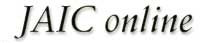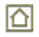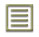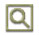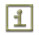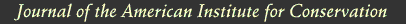JAIC 1987, Volume 26, Number 1, Article 2 (pp. 19 to 25)

### 3 REPORTING OF THE DATA

THE DATA FOR THE peel tests were calculated by averaging the results of the four test pulls. Maximum values were obtained by calculating the means of the highest peaks on the graph read-out and the minimum values were calculated by averaging the lowest points. The average values were determined by calculating the means of the maximum and minimum values. (The smaller values to the right of each number represents the standard deviation).

The lap/shear results were obtained by recording the peaks on each of the four runs and averaging them together. The standard deviations were also calculated.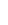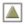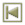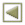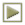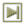Copyright � 1987 American Institute for Conservation of Historic and Artistic Works